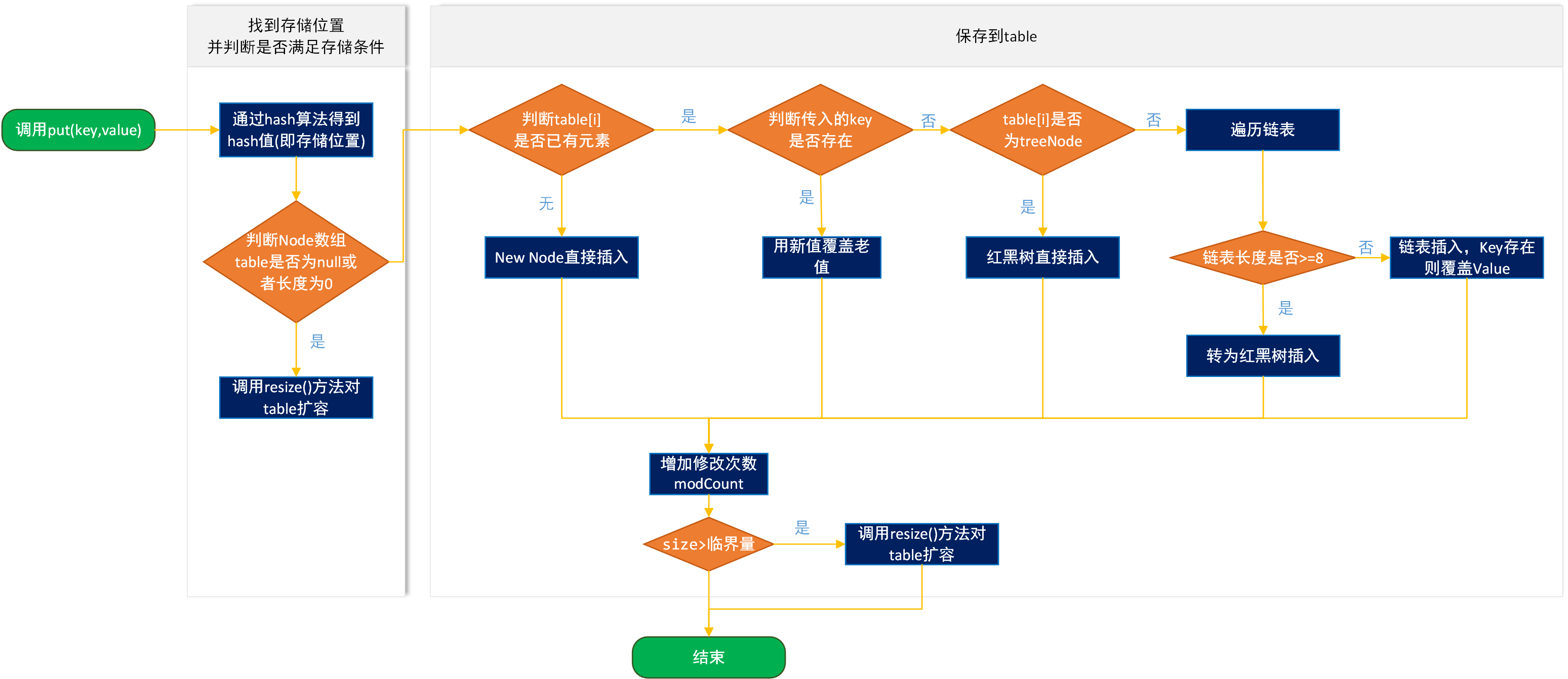# HashMap原理(二) 扩容机制及存取原理

table变量：HashMap的底层数据结构，是Node类的实体数组，用于保存key-value对；

capacity：并不是一个成员变量，但却是一个必须要知道的概念，表示容量；

size变量：表示已存储的HashMap的key-value对的数量；

threshold变量：临界值，当超出该值时，表示table表示该扩容了；

### 一. put方法

HashMap使用哈希算法得到数组中保存的位置，然后调用put方法将key-value对保存到table变量中。我们通过图来演示一下存储的过程。1）通过hash(Object key)算法得到hash值；

2）判断table是否为null或者长度为0，如果是执行resize()进行扩容；

3）通过hash值以及table数组长度得到插入的数组索引i，判断数组table[i]是否为空或为null；
4）如果table[i] == null，直接新建节点添加，转向 8），如果table[i]不为空，转向 5）；
5）判断table[i]的首个元素是否和key一样，如果相同直接覆盖value，这里的相同指的是hashCode以及equals，否则转向 6）；
6）判断table[i] 是否为treeNode，即table[i] 是否是红黑树，如果是红黑树，则直接在树中插入键值对，否则转7）；
7）遍历table[i]，判断链表长度是否大于8，大于8的话把链表转换为红黑树，在红黑树中执行插入操作，否则进行链表的插入操作；遍历过程中若发现key已经存在直接覆盖value即可；
8）插入成功后，判断实际存在的键值对数量size是否超多了最大容量threshold，如果超过，进行扩容。

#### 1. hash方法

static final int hash(Object key) {
int h;
return (key == null) ? 0 : (h = key.hashCode()) ^ (h >>> 16);
}


#### 2. putVal方法

/**
* Implements Map.put and related methods
*
* @param hash hash for key
* @param key the key
* @param value the value to put
* @param onlyIfAbsent if true, don't change existing value
* @param evict if false, the table is in creation mode.
* @return previous value, or null if none
*/
final V putVal(int hash, K key, V value, boolean onlyIfAbsent, boolean evict) {
Node<K,V>[] tab; Node<K,V> p; int n, i;
/**
* 如果当前HashMap的table数组还未定义或者还未初始化其长度，则先通过resize()进行扩容,
* 返回扩容后的数组长度n
*/
if ((tab = table) == null || (n = tab.length) == 0)
n = (tab = resize()).length;
//通过数组长度与hash值做按位与&运算得到对应数组下标，若该位置没有元素，则new Node直接将新元素插入
if ((p = tab[i = (n - 1) & hash]) == null)
tab[i] = newNode(hash, key, value, null);
//否则该位置已经有元素了，我们就需要进行一些其他操作
else {
Node<K,V> e; K k;
//如果插入的key和原来的key相同，则替换一下就完事了
if (p.hash == hash && ((k = p.key) == key || (key != null && key.equals(k))))
e = p;
/**
* 否则key不同的情况下，判断当前Node是否是TreeNode,如果是则执行putTreeVal将新的元素插入
* 到红黑树上。
*/
else if (p instanceof TreeNode)
e = ((TreeNode<K,V>)p).putTreeVal(this, tab, hash, key, value);
//如果不是TreeNode,则进行链表遍历
else {
for (int binCount = 0; ; ++binCount) {
/**
* 在链表最后一个节点之后并没有找到相同的元素，则进行下面的操作，直接new Node插入，
* 但条件判断有可能转化为红黑树
*/
if ((e = p.next) == null) {
//直接new了一个Node
p.next = newNode(hash, key, value, null);
/**
* TREEIFY_THRESHOLD=8,因为binCount从0开始，也即是链表长度超过8（包含）时，
* 转为红黑树。
*/
if (binCount >= TREEIFY_THRESHOLD - 1) // -1 for 1st
treeifyBin(tab, hash);
break;
}
/**
* 如果在链表的最后一个节点之前找到key值相同的（和上面的判断不冲突，上面是直接通过数组
* 下标判断key值是否相同），则替换
*/
if (e.hash == hash &&
((k = e.key) == key || (key != null && key.equals(k))))
break;
p = e;
}
}
if (e != null) { // existing mapping for key
V oldValue = e.value;
//onlyIfAbsent为true时:当某个位置已经存在元素时不去覆盖
if (!onlyIfAbsent || oldValue == null)
e.value = value;
afterNodeAccess(e);
return oldValue;
}
}
++modCount;
//最后判断临界值，是否扩容。
if (++size > threshold)
resize();
afterNodeInsertion(evict);
return null;
}


#### 3. resize方法

HashMap通过resize()方法进行扩容，容量规则为2的幂次

/**
* Initializes or doubles table size.  If null, allocates in
* accord with initial capacity target held in field threshold.
* Otherwise, because we are using power-of-two expansion, the
* elements from each bin must either stay at same index, or move
* with a power of two offset in the new table.
*
* @return the table
*/
final Node<K,V>[] resize() {
Node<K,V>[] oldTab = table;
int oldCap = (oldTab == null) ? 0 : oldTab.length;
int oldThr = threshold;
int newCap, newThr = 0;
//以前的容量大于0，也就是hashMap中已经有元素了，或者new对象的时候设置了初始容量
if (oldCap > 0) {
//如果以前的容量大于限制的最大容量1<<30,则设置临界值为int的最大值2^31-1
if (oldCap >= MAXIMUM_CAPACITY) {
threshold = Integer.MAX_VALUE;
return oldTab;
}
/**
* 如果以前容量的2倍小于限制的最大容量，同时大于或等于默认的容量16，则设置临界值为以前临界值的2
* threshold自然也扩大2倍。
*/
else if ((newCap = oldCap << 1) < MAXIMUM_CAPACITY &&
oldCap >= DEFAULT_INITIAL_CAPACITY)
newThr = oldThr << 1; // double threshold
}
/**
* = tableSizeFor(initialCapacity)， 表示在调用构造器时，默认是将初始容量暂时赋值给了
* threshold临界值，因此此处相当于将上一次的初始容量赋值给了新的容量。什么情况下会执行到这句？当调用
* 了HashMap(int initialCapacity)构造器，还没有添加元素时
*/
else if (oldThr > 0)
newCap = oldThr;
/**
* 调用了默认构造器，初始容量没有设置，因此使用默认容量DEFAULT_INITIAL_CAPACITY（16），临界值
* 就是16*0.75
*/
else {
newCap = DEFAULT_INITIAL_CAPACITY;
}
//对临界值做判断，确保其不为0，因为在上面第二种情况(oldThr > 0)，并没有计算newThr
if (newThr == 0) {
float ft = (float)newCap * loadFactor;
newThr = (newCap < MAXIMUM_CAPACITY && ft < (float)MAXIMUM_CAPACITY ?
(int)ft : Integer.MAX_VALUE);
}
threshold = newThr;

@SuppressWarnings({"rawtypes","unchecked"})
/**构造新表，初始化表中数据*/
Node<K,V>[] newTab = (Node<K,V>[])new Node[newCap];
//将刚创建的新表赋值给table
table = newTab;
if (oldTab != null) {
//遍历将原来table中的数据放到扩容后的新表中来
for (int j = 0; j < oldCap; ++j) {
Node<K,V> e;
if ((e = oldTab[j]) != null) {
oldTab[j] = null;
//没有链表Node节点，直接放到新的table中下标为【e.hash & (newCap - 1)】位置即可
if (e.next == null)
newTab[e.hash & (newCap - 1)] = e;
//如果是treeNode节点，则树上的节点放到newTab中
else if (e instanceof TreeNode)
((TreeNode<K,V>)e).split(this, newTab, j, oldCap);
//如果e后面还有链表节点，则遍历e所在的链表，
else { // 保证顺序
Node<K,V> loHead = null, loTail = null;
Node<K,V> hiHead = null, hiTail = null;
Node<K,V> next;
do {
//记录下一个节点
next = e.next;
/**
* newTab的容量是以前旧表容量的两倍,因为数组table下标并不是根据循环逐步递增
* 的，而是通过（table.length-1）& hash计算得到，因此扩容后，存放的位置就
* 可能发生变化，那么到底发生怎样的变化呢，就是由下面的算法得到.
*
* 通过e.hash & oldCap来判断节点位置通过再次hash算法后，是否会发生改变，如
* 果为0表示不会发生改变，如果为1表示会发生改变。到底怎么理解呢，举个例子：
* e.hash = 13 二进制：0000 1101
* oldCap = 32 二进制：0001 0000
*  &运算：  0  二进制：0000 0000
* 结论：元素位置在扩容后不会发生改变
*/
if ((e.hash & oldCap) == 0) {
if (loTail == null)
else
loTail.next = e;
loTail = e;
}
/**
* e.hash = 18 二进制：0001 0010
* oldCap = 32 二进制：0001 0000
* &运算：  32 二进制：0001 0000
* 结论：元素位置在扩容后会发生改变，那么如何改变呢？
* newCap = 64 二进制：0010 0000
* 通过(newCap-1)&hash
* 即0001 1111 & 0001 0010 得0001 0010，32+2 = 34
*/
else {
if (hiTail == null)
else
hiTail.next = e;
hiTail = e;
}
} while ((e = next) != null);
if (loTail != null) {
loTail.next = null;
/**
* 若(e.hash & oldCap) == 0，下标不变，将原表某个下标的元素放到扩容表同样
* 下标的位置上
*/
}
if (hiTail != null) {
hiTail.next = null;
/**
* 若(e.hash & oldCap) != 0，将原表某个下标的元素放到扩容表中
* [下标+增加的扩容量]的位置上
*/
}
}
}
}
}
return newTab;
}


### 二. get方法

public V get(Object key) {
Node<K,V> e;
//先通过hash(key)找到hash值，然后调用getNode(hash,key)找到节点
return (e = getNode(hash(key), key)) == null ? null : e.value;
}
/**
* Implements Map.get and related methods
*
* @param hash hash for key
* @param key the key
* @return the node, or null if none
*/
final Node<K,V> getNode(int hash, Object key) {
Node<K,V>[] tab; Node<K,V> first, e; int n; K k;
//通过(n - 1) & hash找到数组对应位置上的第一个node
if ((tab = table) != null && (n = tab.length) > 0 &&
(first = tab[(n - 1) & hash]) != null) {
//如果这个node刚好key值相同，直接返回
if (first.hash == hash &&
((k = first.key) == key || (key != null && key.equals(k))))
return first;
//如果不相同就再往下找
if ((e = first.next) != null) {
//如果是treeNode，就遍历红黑树找到对应node
if (first instanceof TreeNode)
return ((TreeNode<K,V>)first).getTreeNode(hash, key);
//如果是链表，遍历链表找到对应node
do {
if (e.hash == hash &&
((k = e.key) == key || (key != null && key.equals(k))))
return e;
} while ((e = e.next) != null);
}
}
return null;
}


### 三. 总结

posted @ 2019-07-08 10:29  工匠初心  阅读(15275)  评论(4编辑  收藏  举报